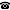# 2014 seminar talk: $\Delta_2$ Degrees without $\Sigma_1$ Induction

Talk held by Wei Li (KGRC) at the KGRC seminar on 2014-03-13.

### Abstract

In this talk, we consider the structure of Turing degrees below $0’$ in the theory that is a fragment of Peano arithmetic without $\Sigma_1$ induction, with special focus on proper $d$-r.e. degrees and non-r.e. degrees. We prove

(1) $P^-+ B\Sigma_1+ \mathrm{Exp}$ implies the existence of a proper $d$-r.e. degree.

(2) Over $P^-+ B\Sigma_1+ \mathrm{Exp}$, the existence of a proper $d$-r.e. degree below $0’$ is equivalent with $I\Sigma_1$.

(3) $P^-+ B\Sigma_1+ \mathrm{Exp}$ is not enough to show there is a non-r.e. degree below $0’$.

Kurt Gödel Research Center for Mathematical Logic. Währinger Straße 25, 1090 Wien, Austria.+43-1-4277-50501. Last updated: 2010-12-16, 04:37.